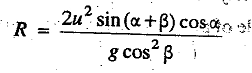# Equation of the Path of a Projectile

Different equations used in projectile motion to calculate time of flight, horizontal range, maximum height etc.

Let
O = Point of projection,
u = Velocity of projection, and
α = Angle of projection with the horizontal.

Consider a point P as the position of particle, after time t seconds with x and y as co-ordinates, as shown in Fig. 1.30.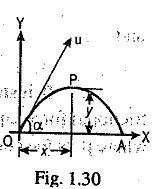The equation of the path of a projectile or the equation of trajectory is given by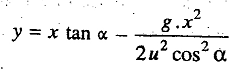Since this is the equation of a parabola, therefore, the path traced by a projectile is a parabola. The following are the important equations used in projectiles:

1. The time of flight (t) of a projectile on a horizontal plane is given by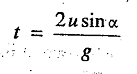2. The horizontal range (R) of a projectile is given by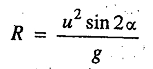For a given velocity of projectile, the range will be maximum when sin 2α = 1 or α=45.

3. The maximum height (H) of a projectile on a horizontal plane is given by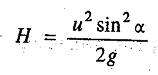4. The time of flight of a projectile when it is projected from O on an upward inclined plane as shown in Fig. 1.31, is given by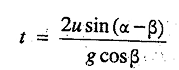where β is the inclination of plane OA with the horizontal.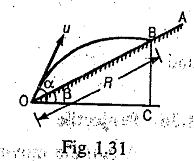When the projectile is projected on a downward inclined plane, then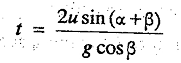5. The range of projectile when it is projected from O to B on an upward inclined plane, as shown in Fig: 1.31, is given by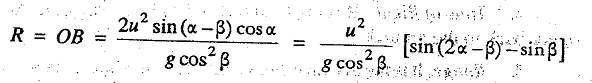For a given velocity of projectile, the range will be maximum when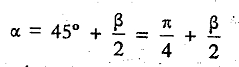When the projectile is projected on a downward inclined plane, then